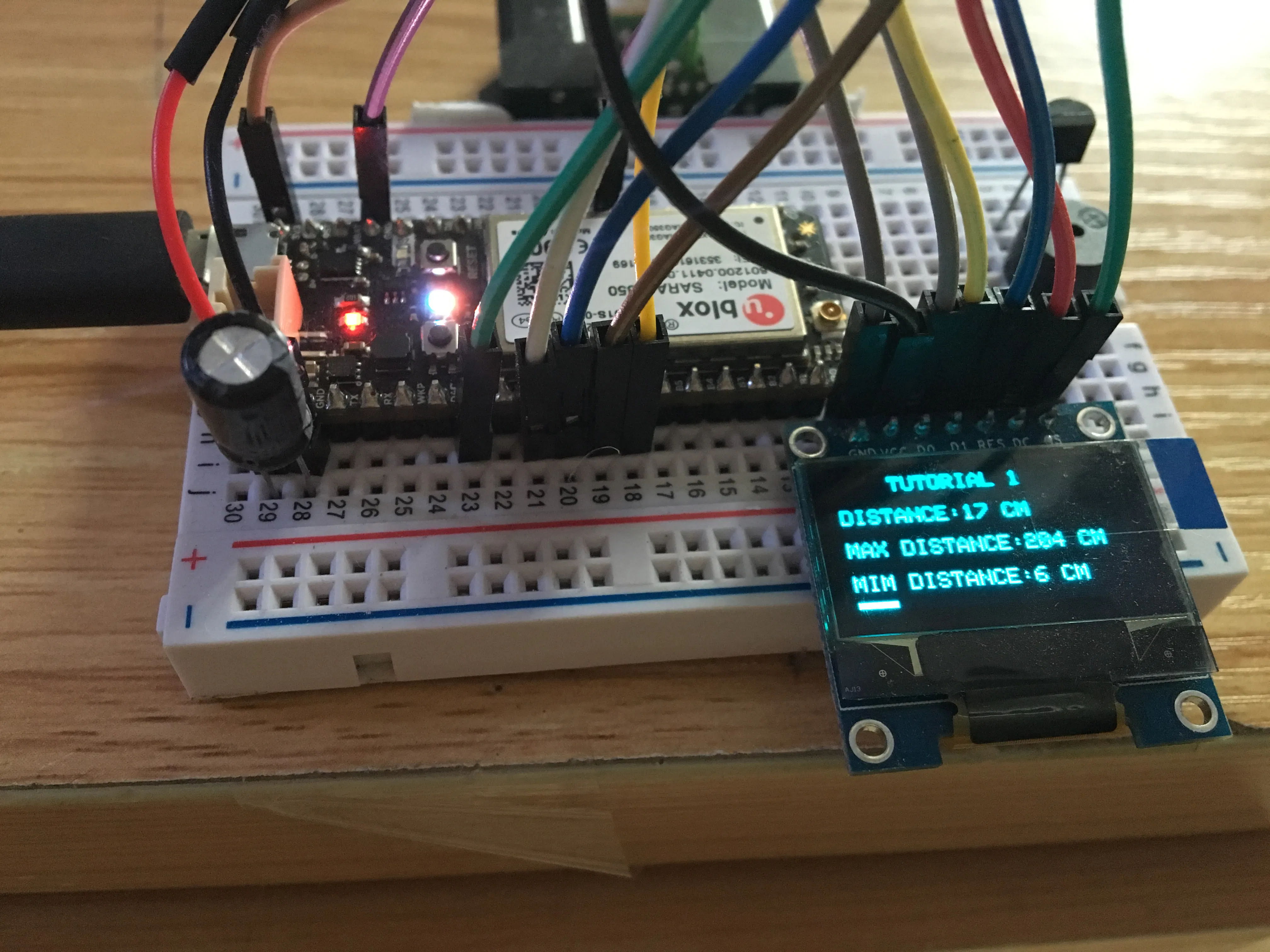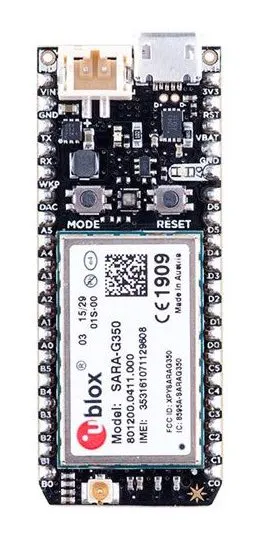# Digital Ruler With IR Distance And Electron

Read out analog value from IR distance sensor and show the transferred distance up on your phone as well as the OLED by electron and IFTTT.

BeginnerShowcase (no instructions)3 hours969## Things used in this project

### Hardware componentsParticle Electron
×1

## Code

### source file

C/C++
Add the library in Particle Build IDE
```// This #include statement was automatically added by the Particle IDE.

#include"application.h"
#include"math.h"
#define IR_PORT  A0

// use hardware SPI,so only need to config DataCommand, ChipSelect, Reset Pins
//#define OLED_MOSI A5  //DI
//#define OLED0_MISO A4
//#define OLED_SCK  A3  //DO
#define OLED_CS A2
#define OLED_DC A1
#define OLED_RESET D0

SYSTEM_MODE(SEMI_AUTOMATIC);

unsigned char distance =10;
unsigned char max_distance = 0;
unsigned char min_distance = 100;

unsigned char algorithm_sensor()
{
unsigned int sensorValue = 0;
unsigned int value = 0;
float analog=0.0;
float temp=0.0;
analog = (sensorValue/4095.0)*3.3;

//get this algorithm from sparkfun web,but seems not very good,
//a better method should be premeasure the adc value at each distance and then use the EXCEL to find out a pow algorithm
//with good parameter.
temp = 306.439 + analog*( -512.611 + analog*(382.268 +analog*(-129.893 + analog*16.2537) ) );
distance = int(round(temp));
Serial.print(distance);
Serial.print("\r\n");
}

void setup() {
Serial.begin(9600);
oled.begin(SSD1306_SWITCHCAPVCC);

Serial.print("This is a demo project for distance sensor\r\n");
pinMode(IR_PORT,INPUT);
delay(2000);
// miniature bitmap display
oled.display();
oled.clearDisplay();
oled.setTextSize(1);
oled.setTextColor(WHITE);

oled.setCursor(0,0);

oled.println("    TUTORIAL 1");     //the first line
oled.println("");       //do nothing here,just to leave one space line
oled.print("DISTANCE:");            //the second line
oled.print(distance, DEC);
oled.println(" CM");
oled.println("");       //do nothing here,just to leave one space line

oled.print("MAX DISTANCE:");      //the third line
oled.print(distance, DEC);
oled.println(" CM");
oled.println("");       //do nothing here,just to leave one space line

oled.print("MIM DISTANCE:");      //the fourth line
oled.print(distance, DEC);
oled.print(" CM");
oled.display();
}

void loop() {

delay(500);                 //update the distance value every 500ms
algorithm_sensor();

if(distance>max_distance)       //get the maximum distance
max_distance = distance;
if(distance<min_distance)       //get the minimum distance
min_distance = distance;

oled.clearDisplay();
oled.setCursor(0,0);        //need to set the original cursor for every loop;

oled.println("    TUTORIAL 1");     //the first line
oled.println("");       //do nothing here,just to leave one space line
oled.print("DISTANCE:");            //the second line
oled.print(distance, DEC);
oled.println(" CM");
oled.println("");       //do nothing here,just to leave one space line

oled.print("MAX DISTANCE:");      //the third line
oled.print(max_distance, DEC);
oled.println(" CM");
oled.println("");       //do nothing here,just to leave one space line

oled.print("MIM DISTANCE:");      //the fourth line
oled.print(min_distance, DEC);
oled.print(" CM");

if(distance>127)
distance = 127;
oled.fillRect(0,60,distance,3,WHITE);       //drawing the distance table.
oled.display();
distance++;
if(distance==100)
distance = 0;
}
```

## Credits

### Eric yuan

5 projects • 1 follower